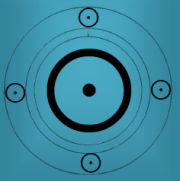Clinton, Chelsea

27 February 1980 at 23:24 (= 11:24 PM ) Little Rock, Arkansas, 34n45, 92w17Rulership, authority, position, advantage Governments, bureaucracies, princely hierarchies, heads of state, paterfamilias.Realisation and fulfilment, Leadership, creation, procreation

Transit On 20.01.2017 at 12:00 PMtSU = KR = SU/VE(Mother) tVE(Mother) = tUR = tPL = SU tJU = tMC(Mother) = MO(Mother) = UR/KR tMO(Mother) = MC(Mother) = VE/KR tKR = tAC = MA/KR = PL = AR/MO tME = tZE = AR = ZE = VE = AC/MA   AR is Aries Point.

Secondary On 20.01.2017 at 12:00 PMpVE = pZE = pJU = VE/ZE = AR/AC = AR/ZE = JU/KR = MN pSU = PMC = SU/MC = SU/JU = AC/KR pKR = pMN = MO/JU = MO/MC = MA pPL = MC/JU = MO/ZE pMO = AC = MC/MN pMA = MO/VE = PL

Secondary Converse On 20.01.2017 at 12:00 PMpVE = pMO = pZE = MO = JU/ZE = MC/ZE pMC = pSU = VE/UR = MC pJU = PL/KR pKR = pMA = SU pPL = AR/MO = PL pME = pUR = pNE = KR = AR/SU = JU/UR pMN = VE

SolarsJU = sVE = sPL = VE/JU = PL/KR sAC = sUR = JU = SU/MO = MA/PL sMO = sKR = sMA = UR/ZE = VE/PL = MA/UR sMC = sSA = VE/ZE = JU/KR = MC/KR sZE = AC = VE = JU/MN sME = MO = MC/SU = VE/MN

LunarpKR = KR = VE/SU pVE = pMC = MC/ZE = JU/ZE = MO pJU = AR/PL pPL = pMN = PL pSU = AC/KR = SU/JU

Lunar Return ProgressedpSU = pKR = SU/VE = KR pMC = pMA = MC/VE = VE/JU pJU = PL pUR = MO = UR = MN/AR pZE = MN/ZE pVE = MO/VE = UR/ZE = AC/UR

Venus ReturnpJU = pMO = pKR = MO = MA/JU = VE/MN = UR pSU = pAC = JU pMA = pUR = UR/ZE = VE/PL pZE = SU/MA = SU/ZE pPL = MN pMN = PL

15.09.2015

Cemal Cicek